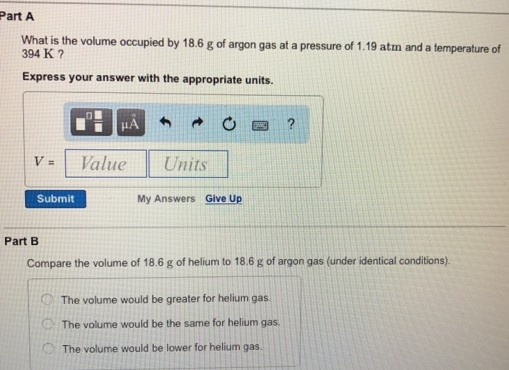# Solution: What is the volume occupied by 18.6 g of argon gas at a pressure of 1.19 atm and a temperature of 394 K? Express your answer with the appropriate units. Compare the volume of 18.6 g of helium to 18.6 g of argon gas (under identical conditions). The volume would be greater for helium gas. The volume would be the same for helium gas. The volume would be lower for helium gas.

###### Problem

What is the volume occupied by 18.6 g of argon gas at a pressure of 1.19 atm and a temperature of 394 K? Express your answer with the appropriate units.

Compare the volume of 18.6 g of helium to 18.6 g of argon gas (under identical conditions).

The volume would be greater for helium gas.

The volume would be the same for helium gas.

The volume would be lower for helium gas.View Complete Written Solution